### Home > A2C > Chapter 12 > Lesson 12.1.4 > Problem12-72

12-72.
1.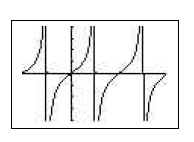Brianna is working on her homework and is asked to state the domain and range for y = tanθ. She enters the equation on her graphing calculator and sets her Xmin to −π and her Xmax to 2π. After looking at its graph, Brianna is still unclear how to state the tangent's domain because of the asymptotes. Homework Help ✎

1. Explain to Brianna the domain of y = tanθ and how to use the graph to make sense of it.

2. The second part of Brianna's problem asked her to graph the transformed equation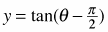. As she entered the equation into her graphing calculator, the screen went blank. (Her batteries were dead.) Explain to Brianna how she can use her first graph to sketch her transformed graph. Include a labeled sketch.

3. Does this transformation have a different domain from that in part (a)? If so, how is it different? What is the range for each function y = tanθ and? Justify your answers completely.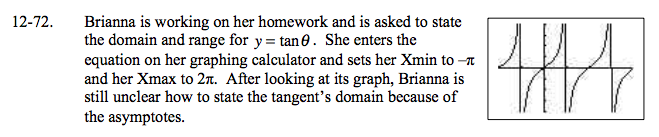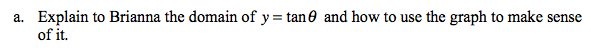Where are the asymptotes located? What does this mean about the domain?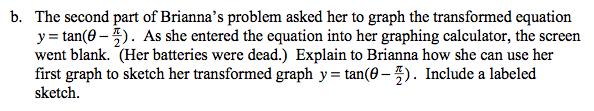$\text{Shift the original graph }\frac{\pi}{2}\text{ radians to the right.}$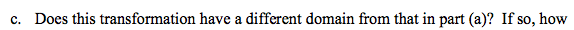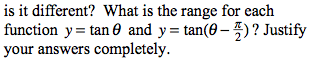How does the transformation affect the positions of the asymptotes?